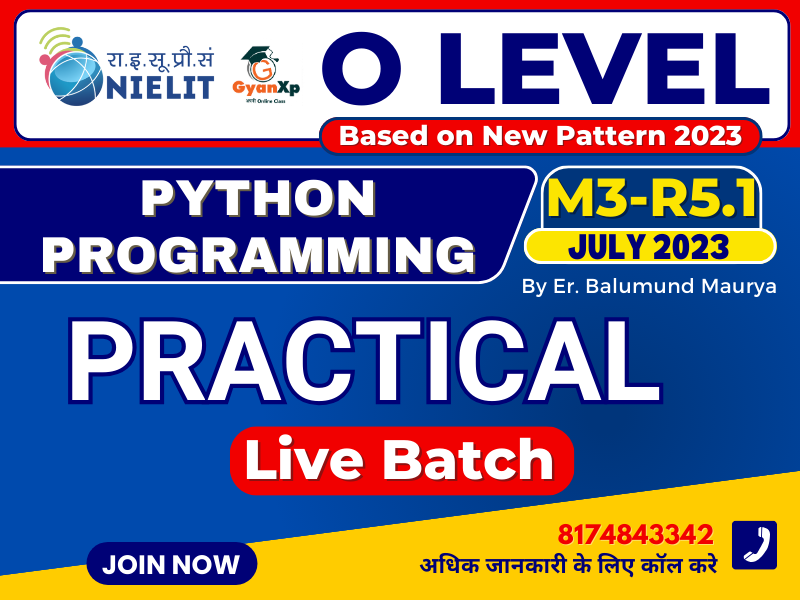## O Level Python Programming (M3-R5.1) Practical Demo

Q1. Write a Python program to multiply two numbers by repeated addition.

Example: 6*3=6+6+6

Q1. Write a program to print multiplication table of a given number

## O Level Practical की बेहरत तैयारी के लिए Join करे Practical Batch